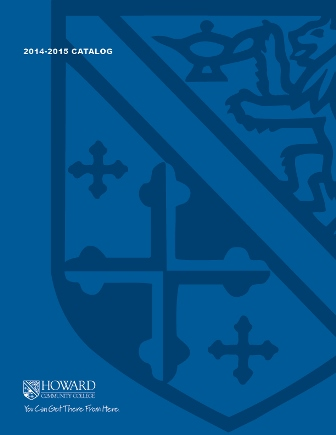2017-2018 College Catalog# MATH 143 Precalculus I

This course can be taken as a single semester course or as the first part of a two semester sequence to prepare for calculus. Topics include polynomial, rational, exponential, and logarithmic functions, an introduction to right and oblique triangle trigonometry and trigonometric functions. Problems will be solved through analytical, numerical and graphical approaches with an emphasis on application problems. Graphing calculators and mathematical software will be employed throughout the course. All sections require the use of the interactive computer program that comes with the software code.

3

### Prerequisite

MATH 070 or appropriate score on mathematics placement test

4 hours weekly

### Course Objectives

1. 1. Communicate mathematical concepts related to precalculus topics using appropriate
symbols, notation, and vocabulary.
2. 2. Construct graphs, tables, and equations of polynomial, power, rational, exponential, logarithmic, and trigonometric functions and describe the characteristics of the functions.
3. 3. Identify and graph shifts, reflections, and transformations of functions.
4. 4. Find inverses of functions algebraically as well as graphically.
5. 5. Apply classic theorems to find the zeros of polynomial equations.
6. 6. Use properties of logarithms and exponents to rewrite and evaluate logarithmic and exponential expressions.
7. 7. Use function models to set up and solve application models.
8. 8. Solve oblique triangles using the Law of Sines and Law of Cosines.
9. 9. Analyze, evaluate, justify, and interpret the reasonableness of solutions.
10. 10. Identify and implement appropriate technologies to efficiently complete tasks that involve solving cross-discipline, mathematically appropriate problems and creating new works to communicate the processes used and solution.

### Course Objectives

1. Communicate mathematical concepts related to precalculus topics using appropriate
symbols, notation, and vocabulary.

2. Construct graphs, tables, and equations of polynomial, power, rational, exponential, logarithmic, and trigonometric functions and describe the characteristics of the functions.

3. Identify and graph shifts, reflections, and transformations of functions.

4. Find inverses of functions algebraically as well as graphically.

5. Apply classic theorems to find the zeros of polynomial equations.

6. Use properties of logarithms and exponents to rewrite and evaluate logarithmic and exponential expressions.

7. Use function models to set up and solve application models.

8. Solve oblique triangles using the Law of Sines and Law of Cosines.

9. Analyze, evaluate, justify, and interpret the reasonableness of solutions.

10. Identify and implement appropriate technologies to efficiently complete tasks that involve solving cross-discipline, mathematically appropriate problems and creating new works to communicate the processes used and solution.